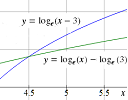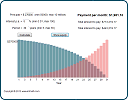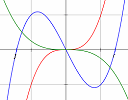Search IntMath
Close

450+ Math Lessons written by Math Professors and Teachers

5 Million+ Students Helped Each Year

1200+ Articles Written by Math Educators and Enthusiasts

Simplifying and Teaching Math for Over 23 Years

# IntMath Newsletter: Solving log equations, graphs

By Murray Bourne, 28 Oct 2013

28 Oct 2013

1. When does log(x − 3) = log x − log 3?
2. Updated math interactives
3. IntMath poll
4. Math puzzles
5. Friday math movie - Smart failure
6. Final thought - Learning to learn

## 1. When does log(x-3) = log x - log 3?A recent comment from a Twitter colleague got me thinking about the value of graphical solutions of equations. When does log(x-3) = log x - log 3? Do you agree with me?

## 2. Updated math interactives

These are the latest conversions I've done from Flash to mobile-friendly javascript.

a. Mortgage calculator with graphThe most expensive thing most people buy is a house. It's a good idea to know what will happen when it comes to the mortgage you'll be paying back for much of your working life. Interactive mortgage calculator

b. Graphing calculatorYou can now save a link to any graph you produce with this calculator. (After you've created your own graph and sized it how you like, a link will appear which you can include in an email or wherever.) Graphing Calculator (SVG)

## 3. IntMath Poll

Generally, how do you feel about school?

It's sad that for many students, school is not a happy place. At least the majority of respondents said either they like it or are neutral.

I hate it and can’t wait to finish 28%

I really love school 26%

It’s OK 22%

I enjoy it 18%

I don’t like it 6%

Current IntMath Poll: With so many students unhappy at school, how can we improve it? You can give your opinion on any (inner) page of IntMath.com.

## 4. Math puzzles

Correct answers with explanation were given by Tomas, Don and Mawanda but my vote for the clearest answer is Mawanda's.

New math puzzle

Find the least 2 positive integers having the remainders 2, 3, 2 when divided by 3, 5, 7 respectively.

## 5. Friday math movie: Smart failure for a fast-changing worldWe don't deal with failure properly in most educational settings. This talk gives some options for improving that. Friday math movie: Smart failure for a fast-changing world

## 6. Final thought: Learning to learn

Most teachers (and parents) tell students to "study hard". But even though some students put a lot of time into study, some produce better outcomes than others.

In a 2011 study, researchers found that if students knew more about their own learning approach, the more likely they were to do well. Also, those who used a variety of learning strategies did better than those who stuck to one or two.

How many of the following successful learning strategies can you say "yes" to?

• I draw pictures or diagrams to help me understand.
• I make up questions that I try to answer.
• When I am learning something new, I think back to what I already know about it.
• I discuss what I am learning with others.
• I practice things over and over until I know them well.
• I think about my thinking, to check if I understand the idea.
• When I don't understand something I go back over it again.
• I make a note of things that I don't understand very wel, so that I can follow them up.
• When I have finished an activity I look back to see how well I did.
• I organize my time to manage my learning.
• I make plans for how to do the activities.

For the ones you said "no" to, why not give them a try?

Until next time, enjoy whatever you learn.

1. Colin Fraser says:

If x has a remainder of 2 when divided by 3 or 7, then x = 21n + 2 for some integer n.
If x has a remainder of 3 when divided by 5, then x = 5m + 3 for some integer m.
Thus, 21n + 2 = 5m + 3, or 21n = 5m + 1.
The smallest n satisfying this equation is 6: 21(6) = 126 = 5(25) + 1.
Therefore x = 126 + 2 = 128, which satisfies the required conditions.

The next largest n to satisfy the equation is 11: 21(11) = 231 = 5(46) + 1.
Therefore, x = 231 + 2 = 233, which also satisfies the required conditions.

In summary, the two integer solutions are 128 and 233.

2. V T John says:

New Maths Puzzle:---: 23 (twenty three) and 233 (two hundred and thirty-three) are the two least integers which leave remainders 2, 3 and 2 when divided by 3, 5 and 7 respectively.

I am aged 74 and my studies of Mathematics ended in March 1958.

Yours sincerely,
V T John

4. Nicos Mavrommatis says:

The integer must have the form x=3k+2, x=5m+3 & x=7n+2. Let w=x-2=3k=7n. Then, 3\w & 7\w -> 21\w because (3,7)=1. Besides, x-3=5m -> (x-2)-1=5m -> w-1=5m -> 5\w-1.
The least w which 21\w is 21 and 5\21-1(=20) simultaneously.
So, the first integer is w=x-2=21 -> x=23.

Next integer (y=w'+2) must have a multiple w'=21k' which has 5\w'-1 respectively. For k'=6, w'=126 and 5\126-1(=125).
So, the second integer is w'=y-2=126 -> y=128.

The least integers are the numbers 23 and 128.

5. hamid zandi says:

a=105*k+23
k=0,1,2,3,...

for example:23,128

### Comment Preview

HTML: You can use simple tags like <b>, <a href="...">, etc.

To enter math, you can can either:

1. Use simple calculator-like input in the following format (surround your math in backticks, or qq on tablet or phone):
a^2 = sqrt(b^2 + c^2)
(See more on ASCIIMath syntax); or
2. Use simple LaTeX in the following format. Surround your math with $$ and $$.
$$\int g dx = \sqrt{\frac{a}{b}}$$
(This is standard simple LaTeX.)

NOTE: You can mix both types of math entry in your comment.